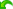MetaEditor 帮助开发程序样板

# 样板

 样板所做的代码布局更改可以撤消。 点击撤销在 编辑 菜单里或按 Ctrl+Z。 样式器支持多种编码标准。可以在编辑器设置中更改样式。下面将详细描述MetaQuotes推荐样式的设计规则。

## 常规

• 用指定内容替换某些行;
• 对齐语言运算符;
• 将注释插入文件标题;
• 在函数、结构、类及其方法之前插入注释;
• 在文件末尾插入注释。

## 空格和空行

### 样板删除空格:

• 在行的末尾;
• 在 ";" 之前;
• "if" 和 "(", "while" 和 "(", "for" 和 "(", "switch" 和 "(", "return" 和 "(" 之间。
• 在 ";" 之后且在 "for()" 之内;
• 在 "!" 之后;
• 在 "(" 和 ")" 附近;
• 在 "<" 和 ">" 附近;
• 在 "|" 和 "^" 附近;
• 在 "+", "-", "/" 和 "%" 附近;
• 在 "[" 和 "]" 附近;
• 在 "==" 和 "==" 附近;
• 在 "!=" 和 "!=" 附近;
• 在 "<=" 和 ">=" 附近;
• 在 "+=" 和 "+=" 附近;
• 在 "-=" 和 "-=" 附近;
• 在 "*=" 和 "*=" 附近;
• 在 "/=" 和 "/=" 附近;
• 在 "<<" 和 ">>" 附近;
• 在 "," 和 "=" 左右侧各一个空格。

### 样板设定:

• 在 "||" 和 "&&" 左右侧各一个空格 (结果: " || " 和 " && ")。

### 其它替换:

• 在 "int* a" 类型的构造器中, 字符 "*" 和 "&" 指的是一个变量而不是类型，所以这个设计被替换为 "int *a";
• 双重空行由一行替换。

void myFunction()
{
if   (I  <  10)
{
printf (“Hello\n”) ;
if (< 5 )
{
printf (“i<5 !\n”) ;
if (< 3)
{
printf (“i<3 !\n”) ;
if (< 2)
{
}
}
}
}
}

void myFunction()
{
if(I<10)
{
printf(“Hello\n”);
if(I<5)
{
printf(“i<5 !\n”);
if(i<3)
{
printf(“i<3 !\n”);
if(i<2)
{
}
}
}
}
}

 无论 MetaEditor 设定 如何，样板的制表符总是等于三个空格。

## 当格式化要考虑行间对齐时则排除替换

• "//" ― С 风格的注释符号;
• "{" ― 开口大括号;
• "}" ― 封口大括号;
• "return" ― "return" 操作符;
• "delete" ― "delete" 操作符;
• "=" ― 等号;
• ":" ― 冒号;
• "." ― 句号。

## 格式化函数和操作符

• 在函数/操作符类型声明的开口和封口 "{" 和 "}" 符号之前加入两个空格。
• 位于函数/操作符的 "{" 和 "}" 符号之间的每一行，操作符开始之前均放置三个空格。
• 在未封口 "{" 或 "}" 符号的同一行内有文本情况下, 这些符号和文本会被整理到不同的行。
• 考虑到前一行的内容，也执行对齐。 如果括号未封闭，或者算术表达式在前一行中尚未完整，则当前行从前一行的 "(" 或 "=" 的第一个位置开始。
• 条件分支 "else" 总是设定与 "if" 相同。

void myFunction()  {
int k,t=0;
for(int i=0;i<10;i++){
k[i]=i;
t+=i;}
if  (button==name) {
bool state=ObjectGetInteger(0,name,OBJPROP_STATE);
if(!state)
ObjectSetInteger(0,name,OBJPROP_STATE,false);}
else {
ObjectSetInteger(0,name,OBJPROP_STATE,false);}
}

void myFunction()
{
int k,t=0;
for(int i=0;i<10;i++)
{
k[i]=i;
t+=i;
}
if(button==name)
{
bool state=ObjectGetInteger(0,name,OBJPROP_STATE);
if(!state)
ObjectSetInteger(0,name,OBJPROP_STATE,false);
}
else
{
ObjectSetInteger(0,name,OBJPROP_STATE,false);
}
}

## 当声明结构、类和枚举时进行对齐

• 声明成员/方法的指定类型之前放置三个空格;
• 声明结构或类的成员及方法时，其名称要从每一行的第 22 个字符位开始指定;
• 访问成员和方法的定域符 ("private", "protected", "public" 和 "virtual") 要与 "class" 保留字对齐。

private:
int m_rows;
int m_columns;
int m_button_width;
int m_button_height;
int m_top;
int m_left;
};

{
private:
int               m_rows;
int               m_columns;
int               m_button_width;
int               m_button_height;
int               m_top;
int               m_left;
};

## 注释对齐

• 函数或方法定义中的第一级注释与左侧对齐（没有缩进）;
• 声明中的第一级注释与每行的第三个字符位对齐;
• 随后级别的注释与它们所处操作符的相应级别等同。

void myFunction()  {
//--- 第一级注释
int k,t=0;
for(int i=0;i<10;i++){
k[i]=i;
t+=i;}
if  (button==name) {
bool state=ObjectGetInteger(0,name,OBJPROP_STATE);
//--- 第二级注释
if(!state)
ObjectSetInteger(0,name,OBJPROP_STATE,false);}
else {
ObjectSetInteger(0,name,OBJPROP_STATE,false);}
}

void myFunction()
{
//--- 第一级注释
int k,t=0;
for(int i=0;i<10;i++)
{
k[i]=i;
t+=i;
}
if(button==name)
{
bool state=ObjectGetInteger(0,name,OBJPROP_STATE);
//--- 第二级注释
if(!state)
ObjectSetInteger(0,name,OBJPROP_STATE,false);
}
else
{
ObjectSetInteger(0,name,OBJPROP_STATE,false);
}
}

{
private:
int m_rows;
//--- 行数
int m_columns;
//--- 列数
int m_button_width;
//--- cell width

};

{
private:
int               m_rows;
//--- 行数
int               m_columns;
//--- 列数
int               m_button_width;
//--- 单元宽度

};

## 插入注释

 //+------------------------------------------------------------------+ //|                                                      ProjectName | //|                                    Copyright © 2009, CompanyName.| //|                                       http://www.companyname.net | //+------------------------------------------------------------------+

 //+------------------------------------------------------------------+ //|                                                                  | //+------------------------------------------------------------------+

 //+------------------------------------------------------------------+